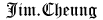# 4clojure Notes

(solutions and notes when I worked on 4clojure problems)

## Difficulty: Elementary

16. Hello World

the most straightforward is using `str`:

``#(str "Hello, " % "!")``

but I found immo's solution:

``format "Hello, %s!"``

is simple too (not a function as required, but it passes the unit tests :D)

72. Rearranging Code: ->>

also the solution is simple: `reduce +`

but `apply +` also works.

156. Map Defaults

my solution:

``#(apply hash-map (interleave %2 (repeat %1)))``

other solutions:

when transforming from one collection type to another, can always use `reduce` (austintaylor's solution):

``(fn [x s] (reduce #(assoc %1 %2 x) {} s))``

and immo's neat solution:

``#(zipmap %2 (repeat %))``

## Difficulty: Easy

19. Last Element

my solution: `(comp first reverse)`

also works: `#(first (reverse %))` and `#(-> % reverse first)`

learned that when there's only one argument passing to each function, use `comp`

another interesting solution is `(comp peek vec)` by immo.

22. Count a Sequence

my solution: `#(reduce + (map (constantly 1) %))`

and cheated solution from immo: `#(.size (vec %))`

26. Fibonacci Sequence

my solution:

``````(fn [n]
(take n``````

(map first (iterate (fn [[x y]] [y (+ x y)]) [1 1]))))

the best is _pcl's solution:

``````;; TDD principle: Write the minimum amount of code required to make the test pass :)
(fn [i] (take i '(1 1 2 3 5 8 13 21))) ``````

28. Flatten a Sequence

my solution:

``(fn f [s] (if (sequential? s) (mapcat f s) (list s)))``

however, others are using `coll?` instead of `sequential?`

32. Duplicate a Sequence

my solution:

``(fn [s] (reduce (fn [acc x] (conj acc x x)) [] s)) ``

the best is `#(interleave % %)`

another one is `mapcat #(list % %)`

38. Maximum value

my solution:

``(fn [& n] (reduce (fn [x acc] (if (> x acc) x acc)) 0 n))``

not good, I know, especially comparing to immo's

``#(last (sort %&))``